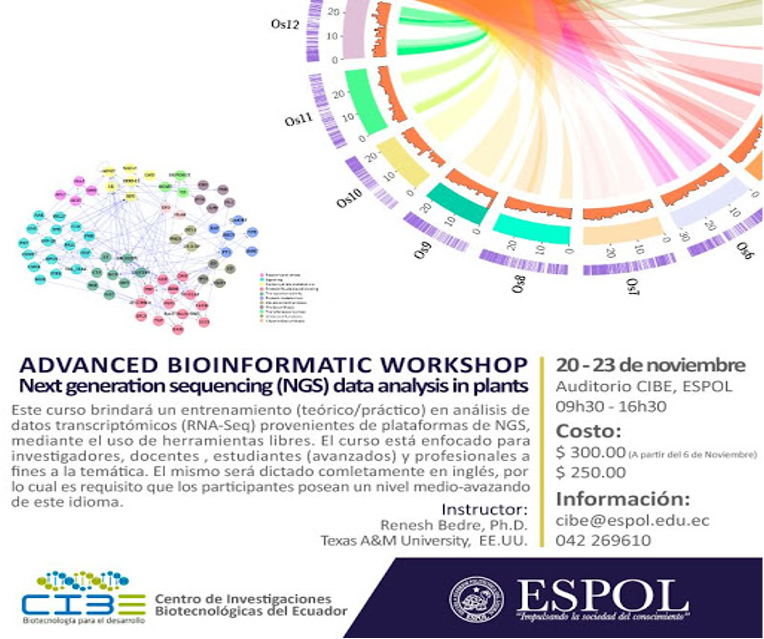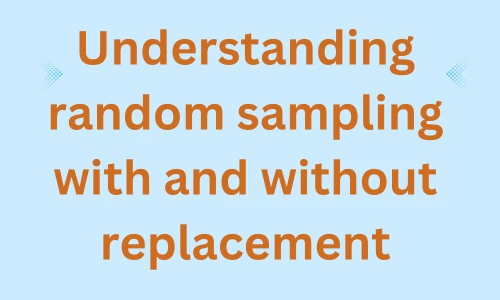# Make discoveries with your data

## Subscribe to get new article to your email when published

* indicates required

### Recent posts## Correlation analysis in Python

Correlation analysis using Python code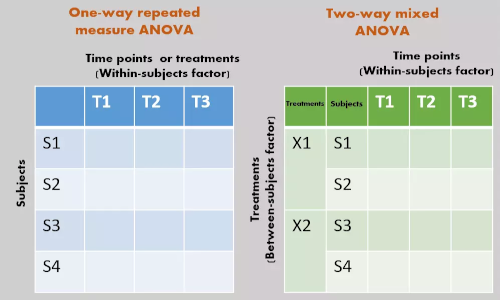## Repeated Measures ANOVA using Python and R (with examples)

Repeated Measure ANOVA in Python and R. This article explains repeated Measure ANOVA model, multiple pairwise comparisons, and results interpretation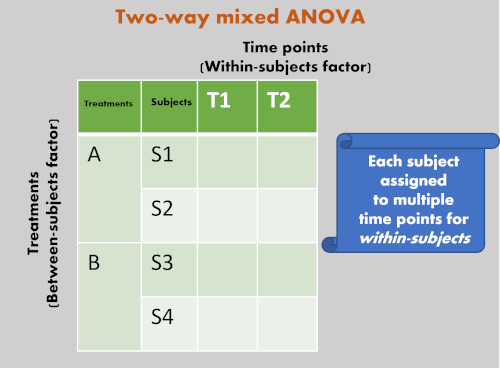## Mixed ANOVA using Python and R (with examples)

Learn to perform mixed ANOVA, check assumptions, and post-hoc tests for significant interactions and main effects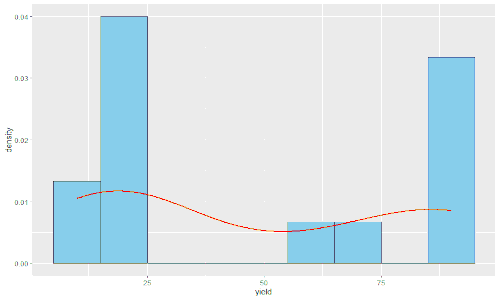## Kruskal-Wallis test in R [with example and code]

Kruskal-Wallis test is a non-parametric test for estimating the differences between multiple groups. Learn the basics of Kruskal-Wallis test, its underlying ...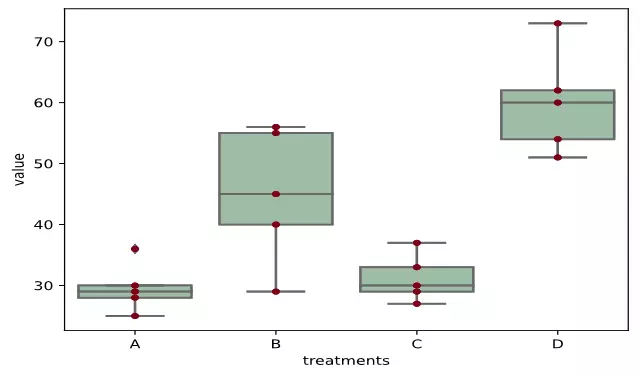## ANOVA using Python (with examples)

One and two-way ANOVA in Python. This article explains ANOVA model, tables, formula, calculation, multiple pairwise comparisons, and results interpretation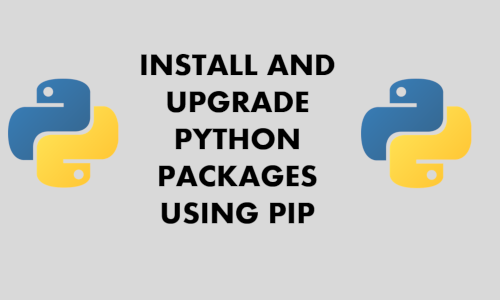## Install and upgrade Python packages using pip, conda, and virtual environment on Windows, Linux, and macOS

Learn how to install, upgrade, and check versions of Python packages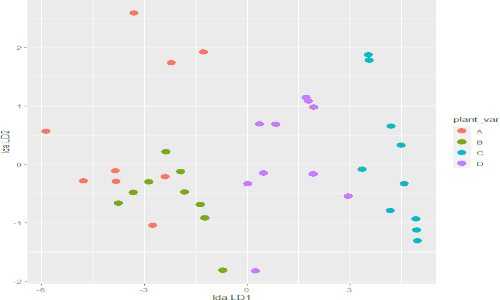## MANOVA using R (with examples and code)

A step-by-step article for performing MANOVA in R. This article describes the background of MANOVA, assumptions, hypotheses, and codes for performing MANOVA ...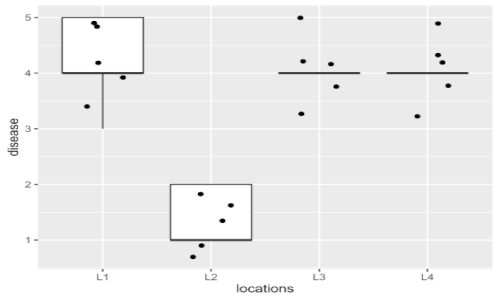## Friedman test using R (with examples and code)

A step-by-step article for performing Friedman test in R## Friedman test using Python (with examples and code)

A step-by-step article for performing Friedman test in Python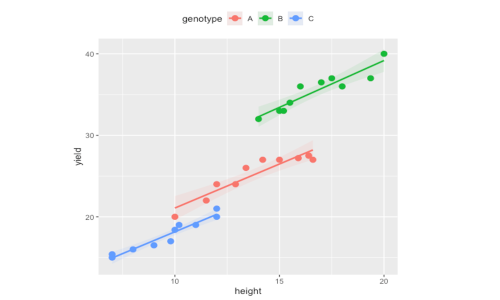## ANCOVA using R and Python (with examples and code)

A step-by-step article for performing ANCOVA in R and Python. This article describes the background of ANCOVA, assumptions, hypotheses, and codes for perform...